#雪月书韵茶香

Java 流程控制语句
/

## Java 流程控制语句# 本文内容

• if else 判断语句
• switch 选择语句
• for 循环语句
• while 循环语句
• do while 循环语句
• 跳出语句 break ，continue

# 第一章 流程控制

## 1.2 顺序结构

``````public static void main(String[] args){
//顺序执行，根据编写的顺序，从上到下运行
System.out.println(1);
System.out.println(2);
System.out.println(3);
}
``````

# 第二章 判断语句

## 2.1 判断语句 1--if

• if 语句第一种格式： if

if(关系表达式)｛

• 执行流程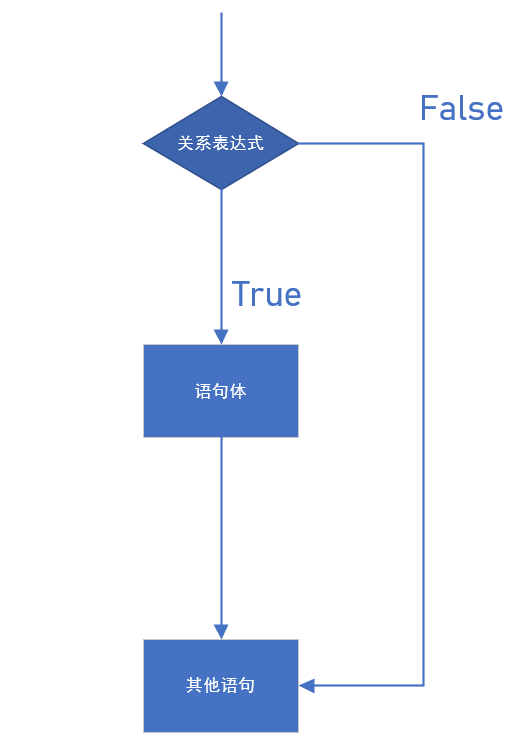``````public static void main(String[] args){
System.out.println("开始");
// 定义两个变量
2.2  判断语句2--if...else
if 语句第二种格式： if...else

int a = 10;
int b = 20;
//变量使用if判断
if (a == b){
System.out.println("a等于b");
}
int c = 10;
if(a == c){
System.out.println("a等于c");
}
System.out.println("结束");
｝
``````

## 2.2 判断语句 2--if...else

• if 语句第二种格式： if...else

if(关系表达式) {

}else {

}

• 执行流程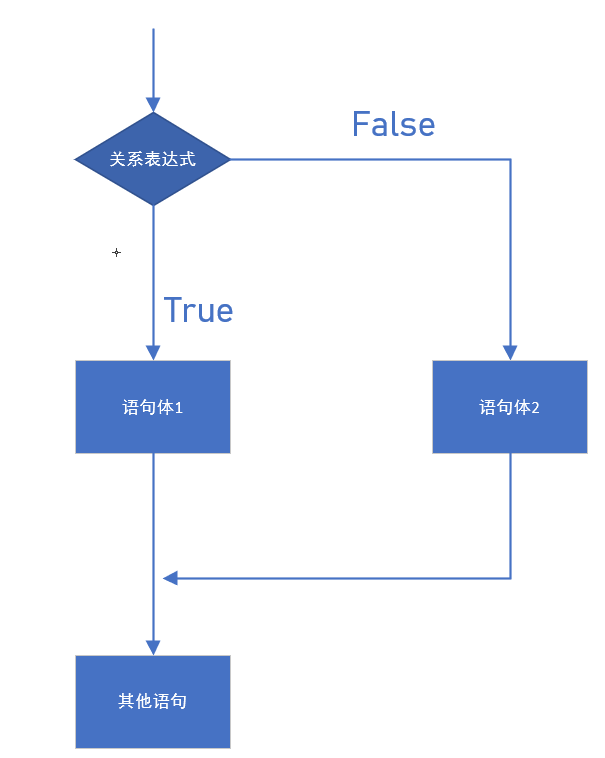``````public static void main(String[] args){
// 判断给定的数据是奇数还是偶数
// 定义变量
int a = 1;
if(a % 2 == 0) {
System.out.println("a是偶数");
} else{
System.out.println("a是奇数");
}
System.out.println("结束");
}
``````

## 2.3 判断语句 3--if..else if...else

• if 语句第三种格式： if...else if ...else

if (判断条件 1) {

} else if (判断条件 2) {

}
...
}else if (判断条件 n) {

} else {

}

• 执行流程

...``````public static void main(String[] args) {
// x和y的关系满足如下：
// x>=3 y = 2x + 1;
//‐1<=x<3 y = 2x;
// x<=‐1 y = 2x – 1;
// 根据给定的x的值，计算出y的值并输出。
// 定义变量
int x = 5;
int y;
if (x>= 3) {
y = 2 * x + 1;
} else if (x >= ‐1 && x < 3) {
y = 2 * x;
} else  {
y = 2 * x ‐ 1;
}
System.out.println("y的值是："+y);
}
``````

## 2.4 语句练习

``````public static void main(String[] args) {
int score = 100;
if(score<0 || score>100){
System.out.println("你的成绩是错误的");
}else if(score>=90 && score<=100){
System.out.println("你的成绩属于优秀");
}else if(score>=80 && score<90){
System.out.println("你的成绩属于好");
}else if(score>=70 && score<80){
System.out.println("你的成绩属于良");
}else if(score>=60 && score<70){
System.out.println("你的成绩属于及格");
}else {
System.out.println("你的成绩属于不及格");
}
}}
``````

## 2.5 if 语句和三元运算符的互换

``````public static void main(String[] args) {
int a = 10;
int b = 20;
//定义变量，保存a和b的较大值
int c;
if(a > b) {
c = a;
} else {
c = b;
}
//可以上述功能改写为三元运算符形式
c = a > b ? a:b;
}
``````

# 第三章 选择语句

## 3.1 选择语句--switch

• switch 语句格式：
``````switch(表达式) {
case 常量值1:
语句体1;
break;
case 常量值2:
语句体2;
break;
...
default:
语句体n+1;
break;
}
``````
• 执行流程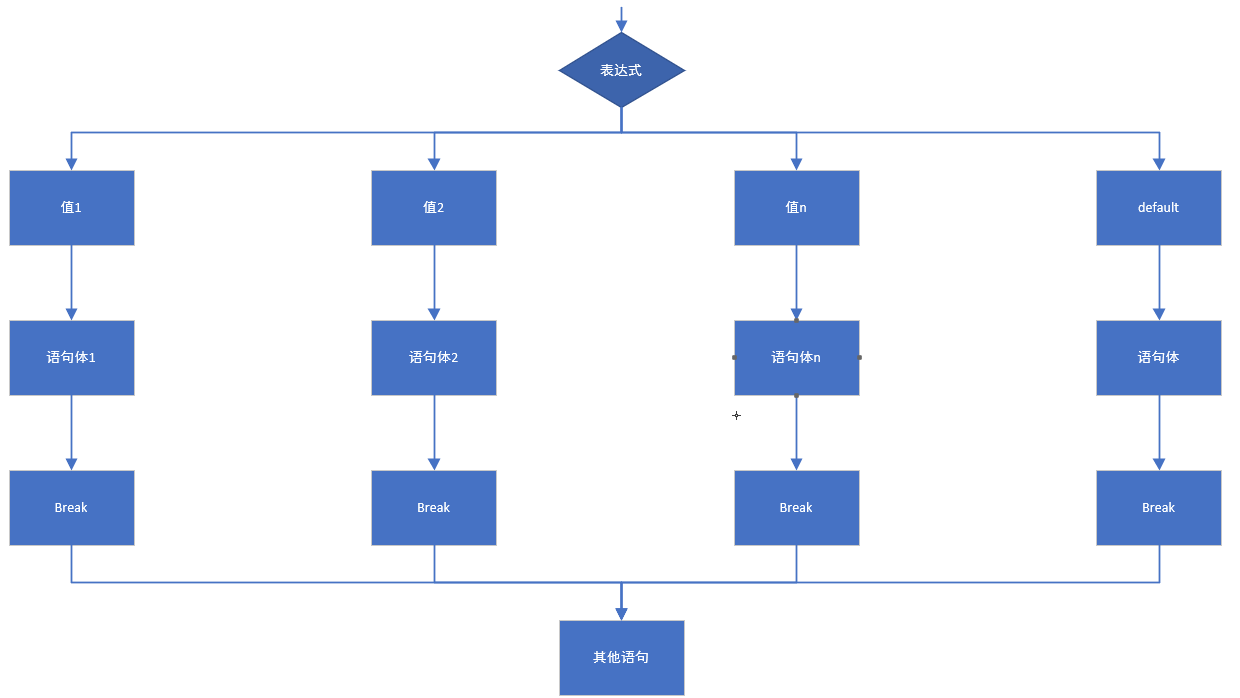``````public static void main(String[] args) {
//定义变量，判断是星期几
int weekday = 6;
//switch语句实现选择
switch(weekday) {
case 1:
System.out.println("星期一");
break;
case 2:
System.out.println("星期二");
break;
case 3:
System.out.println("星期三");
break;
case 4:
System.out.println("星期四");
break;
case 5:
System.out.println("星期五");
break;
case 6:
System.out.println("星期六");
break;
case 7:
System.out.println("星期日");
break;
default:
System.out.println("你输入的数字有误");
break;
}
}
``````

switch 语句中，表达式的数据类型，可以是 byte，short，int，char，enum（枚举），JDK7 后可以接收字符串

## 3.2 case 的穿透性

``````
public static void main(String[] args) {
int i = 5;
switch (i){
case 0:
System.out.println("执行case0");
break;
case 5:
System.out.println("执行case5");
case 10:
System.out.println("执行case10");
default:
System.out.println("执行default");
}
}
``````

# 第四章 循环语句

## 4.2 循环语句 1--for

• for 循环语句格式：

for(初始化表达式 ① ; 布尔表达式 ② ; 步进表达式 ④ ){

}

• 执行流程

① 负责完成循环变量初始化

② 负责判断是否满足循环条件，不满足则跳出循环

③ 具体执行的语句

④ 循环后，循环条件所涉及变量的变化情况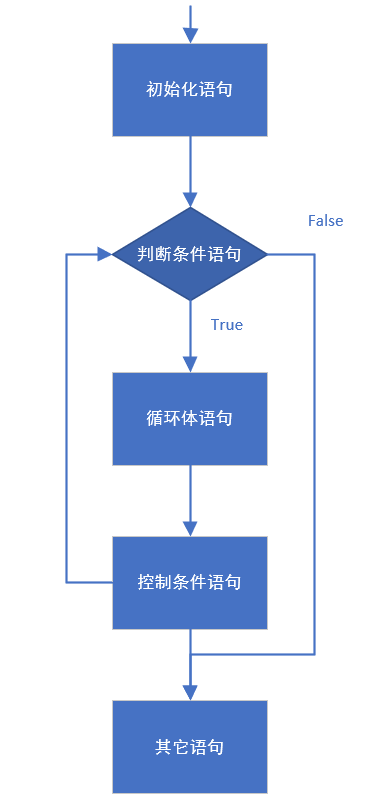``````public static void main(String[] args) {
//控制台输出10次HelloWorld，不使用循环
System.out.println("HelloWorld");
System.out.println("HelloWorld");
System.out.println("HelloWorld");
System.out.println("HelloWorld");
System.out.println("HelloWorld");
System.out.println("HelloWorld");
System.out.println("HelloWorld");
System.out.println("HelloWorld");
System.out.println("HelloWorld");
System.out.println("‐‐‐‐‐‐‐‐‐‐‐‐‐‐‐‐‐‐‐‐‐‐‐‐‐");
//用循环改进，循环10次
//定义变量从0开始，循环条件为<10
for(int x = 0; x < 10; x++) {
System.out.println("HelloWorld"+x);
}
}
``````
• 循环练习：使用循环，计算 1-100 之间的偶数和
``````
public static void main(String[] args) {
//1.定义一个初始化变量,记录累加求和,初始值为0
int sum = 0;
//2.利用for循环获取1‐100之间的数字
for (int i = 1; i <= 100; i++) {
//3.判断获取的数组是奇数还是偶数
if(i % 2==0){
//4.如果是偶数就累加求和
sum += i;
}
}
//5.循环结束之后,打印累加结果
System.out.println("sum:"+sum);
}
``````

## 4.3 循环语句 2--while

• while 循环语句格式

while(布尔表达式 ② ){

}

• 执行流程

① 负责完成循环变量初始化

② 负责判断是否满足循环条件，不满足则跳出循环

③ 具体执行的语句

④ 循环后，循环变量的变化情况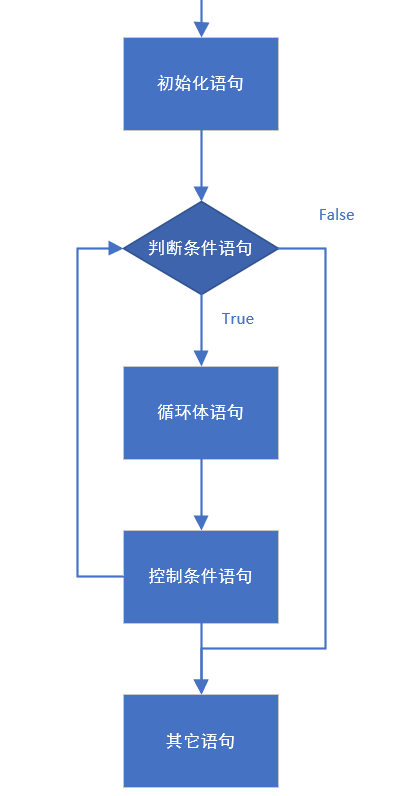while 循环输出 10 次 HelloWorld

``````
public static void main(String[] args) {
//while循环实现打印10次HelloWorld
//定义初始化变量
int i = 1;
//循环条件<=10
while(i<=10){
System.out.println("HelloWorld");
//步进
i++;
}
}
``````

while 循环计算 1-100 之间的和

``````public static void main(String[] args) {
//使用while循环实现
//定义一个变量,记录累加求和
int sum = 0;
//定义初始化表达式
int i = 1;
//使用while循环让初始化表达式的值变化
while(i<=100){
//累加求和
sum += i ;
//步进表达式改变变量的值
i++;
}
//打印求和的变量
System.out.println("1‐100的和是："+sum);
}
``````

## 4.4 循环语句 3--do...while

• do...while 循环格式

do{

}while(布尔表达式 ② );

• 执行流程

① 负责完成循环变量初始化

② 负责判断是否满足循环条件，不满足则跳出循环

③ 具体执行的语句

④ 循环后，循环变量的变化情况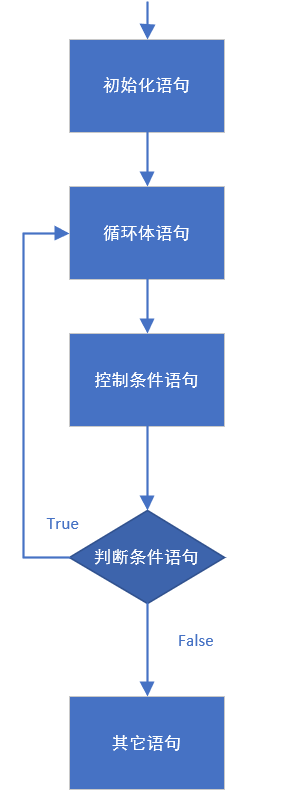``````public static void main(String[] args) {
int x=1;
do {
System.out.println("HelloWorld");
x++;
}while(x<=10);
}
``````

do...while 循环的特点：无条件执行一次循环体，即使我们将循环条件直接写成 false，也依然会循环一次。这样的

``````public static void main(String[] args){
do{
System.out.println("无条件执行一次");
}while(false);
}
``````

## 4.5 循环语句的区别

### break

• 使用场景：终止 switch 或者循环

``````
public static void main(String[] args) {
for (int i = 1; i<=10; i++) {
//需求:打印完两次HelloWorld之后结束循环
if(i == 3){
break;
}
System.out.println("HelloWorld"+i);
}
}
``````

### continue

• 使用场景：结束本次循环，继续下一次的循环
``````
public static void main(String[] args) {
for (int i = 1; i <= 10; i++) {
//需求:不打印第三次HelloWorld
if(i == 3){
continue;
}
System.out.println("HelloWorld"+i);
}
}
``````

# 第五章 扩展知识点

## 5.1 死循环

• 死循环： 也就是循环中的条件永远为 true，死循环的是永不结束的循环。例如：while(true){}

## 5.2 嵌套循环

• 所谓嵌套循环 ，是指一个循环的循环体是另一个循环。比如 for 循环里面还有一个 for 循环，就是嵌套循环。总共的循环次数 = 外循环次数*内循环次数
• 嵌套循环格式

for(初始化表达式 ① ; 循环条件 ② ; 步进表达式 ⑦ ) {
for(初始化表达式 ③ ; 循环条件 ④ ; 步进表达式 ⑥ ) {

}
}

• 嵌套循环执行流程

• 练习:使用嵌套循环，打印 5*8 的矩
``````public static void main(String[] args) {
//5*8的矩形，打印5行*号，每行8个
//外循环5次，内循环8次
for(int i = 0; i < 5; i++){
for(int j = 0; j < 8; j++){
//不换行打印星号
System.out.print("*");
}
//内循环打印8个星号后，需要一次换行
System.out.println();
}
｝
``````